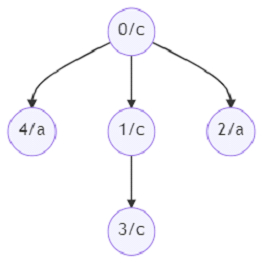# Program to find number of nodes in the sub-tree with the same label using Python

Suppose we have a rooted general tree with n nodes, whose nodes are numbered from 0 to n-1. Each node has a label with lowercase English letter. The labels are given as input in labels array, where lables[i] contains label for ith node. The tree is represented by edge list where each edge e has [u,v] represents u is parent and v is child. We have to find an array A of size n, represents number of nodes in the subtree of the ith node with same label as i

So, if the input is likeHere n = 5 and label = “ccaca”

then the output will be [3, 2, 1, 1, 1] as the root has three descendants with same label, node 1 has two descendants, and all other itself holding that label.

To solve this, we will follow these steps −

• E := Make graph from the given edge list

• N := a map containing each node number and its corresponding label

• R := a list of size n and fill with 0

• Define a function r() . This will take ni

• C := a map to hold frequency of a key

• for each e in E[ni], do

• delete ni from E[e]

• update r(e) in C

• update N[ni] in C

• R[ni] := C[N[ni]]

• return C

• From the main method call r(0)

• return R

Let us see the following implementation to get better understanding −

## Example

Live Demo

from collections import defaultdict, Counter
def solve(n, edges, labels):
E = defaultdict(set)
for f,t in edges:
N = {i:e for i,e in enumerate(labels)}
R = *n
def r(ni):
C = Counter()
for e in E[ni]:
E[e].remove(ni)
C.update(r(e))
C.update((N[ni]))
R[ni] = C[N[ni]]
return C
r(0)
return R
n = 5
edges = [[0,1],[0,2],[1,3],[0,4]]
labels = "ccaca"
print(solve(n, edges, labels))

## Input

5, [[0,1],[0,2],[1,3],[0,4]], "ccaca"

## Output

[3, 2, 1, 1, 1]

Updated on: 29-May-2021

322 Views